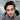# 0.1 + 0.2 = 0.30000000000000004?

November 10, 2019

Try to run the following code in your browser console

``console.log(0.1 + 0.2);``

What do you see?

A freaking 0.30000000000000004?

But why?

A number is stored in memory in its binary form, a sequence of bits – ones and zeroes. But fractions like 0.1, 0.2 that look simple in the decimal numeric system are actually unending fractions in their binary form.
In other words, what is 0.1? It is one divided by ten 1/10, one-tenth. In decimal numeral system such numbers are easily representable. Compare it to one-third: 1/3. It becomes an endless fraction 0.33333(3).
So, division by powers 10 is guaranteed to work well in the decimal system, but division by 3 is not. For the same reason, in the binary numeral system, the division by powers of 2 is guaranteed to work, but 1/10 becomes an endless binary fraction.
There’s just no way to store exactly 0.1 or exactly 0.2 using the binary system, just like there is no way to store one-third as a decimal fraction.
The numeric format IEEE-754 solves this by rounding to the nearest possible number. These rounding rules normally don’t allow us to see that “tiny precision loss”, but it exists.

### ResoucesWritten by Pawan Kumar who lives and works in New Delhi as a Full Stack developer.You should follow him on Twitter